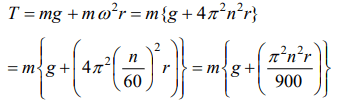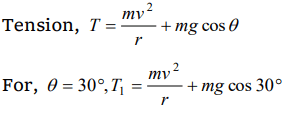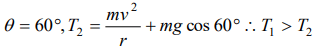## Motion in a Plane Questions and Answers Part-15

1. A stone tied with a string, is rotated in a vertical circle. The minimum speed with which the string has to be rotated
a) Is independent of the mass of the stone
b) Is independent of the length of the string
c) Decreases with increasing mass of the stone
d) Decreases with increasing in length of the string

Explanation: Is independent of the mass of the stone

2. For a particle in a non-uniform accelerated circular motion
a) Velocity is radial and acceleration is transverse only
b) Velocity is transverse and acceleration is radial only
c) Velocity is radial and acceleration has both radial and transverse components
d) Velocity is transverse and acceleration has both radial and transverse components

Explanation: In non-uniform circular motion particle possess both centripetal as well as tangential acceleration.

3. A fighter plane is moving in a vertical circle of radius ‘r’. Its minimum velocity at the highest point of the circle will be
a) $\sqrt{3 g r}$
b) $\sqrt{2 g r}$
c) $\sqrt{ g r}$
d) $\sqrt{ g r\diagup2}$

Explanation: $\sqrt{ g r}$

4. A ball is moving to and fro about the lowest point A of a smooth hemispherical bowl. If it is able to rise up to a height of 20 cm on either side of A, its speed at A must be ($Take = 10m\diagup s^{2}$  , mass of the body = 5g)
a) 0.2 m/s
b) 2 m/s
c) 4 m/s
d) 4.5 ms–1

Explanation:5. A stone of mass m is tied to a string and is moved in a vertical circle of radius r making n revolutions per minute. The total tension in the string when the stone is at its lowest point is
a) mg
b) $m \left(g+\pi nr^{2}\right)$
c) $m \left(g+\pi nr\right)$
d) $m \left\{g+\left(\pi^{2}n^{2}r\right)\right/900\}$

Explanation:6. A coin, placed on a rotating turn-table slips, when it is placed at a distance of 9 cm from the centre. If the angular velocity of the turn-table is trippled, it will just slip, if its distance from the centre is
a) 27 cm
b) 9 cm
c) 3 cm
d) 1 cm

Explanation:7. When a ceiling fan is switched off its angular velocity reduces to 50% while it makes 36 rotations. How many more rotation will it make before coming to rest (Assume uniform angular retardation)
a) 18
b) 12
c) 36
d) 48

Explanation:8. A body crosses the topmost point of a vertical circle with critical speed. Its centripetal acceleration, when the string is horizontal will be
a) 6 g
b) 3 g
c) 2 g
d) g

Explanation:9. A simple pendulum oscillates in a vertical plane. When it passes through the mean position, the tension in the string is 3 times the weight of the pendulum bob. What is the maximum displacement of the pendulum of the string with respect to the vertical
a) $30^{\circ}$
b) $45^{\circ}$
c) $60^{\circ}$
d) $90^{\circ}$10. A particle is moving in a vertical circle. The tensions in the string when passing through two positions at angles 30o and 60o from vertical (lowest position) are $T_{1} and T_{2}$  respectively. then
a) $T_{1}= T_{2}$
b) $T_{2}> T_{1}$
c) $T_{1}> T_{2}$